Year 7 Interactive Maths - Second Edition

## Bearings

A directional compass is shown below.  It is used to find a direction or bearing .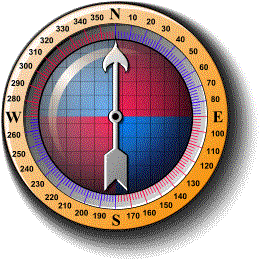The four main directions of a compass are known as cardinal points.  They are north (N), east (E), south (S) and west (W).  Sometimes, the half-cardinal points of north-east (NE), north-west (NW), south-east (SE) and south-west (SW) are shown on the compass.  The above compass shows degree measurements from 0° to 360° in 10° intervals with:

• north representing 0° or 360°
• east representing 90°
• south representing 180°
• west representing 270°

When using a directional compass, hold the compass so that the point marked north points directly away from you.  Note that the magnetic needle always points to the north.

### Bearing

The true bearing to a point is the angle measured in degrees in a clockwise direction from the north line.  We will refer to the true bearing simply as the bearing.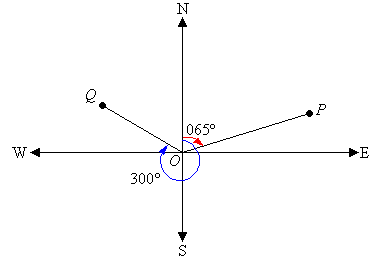For example, the bearing of point P is 065º which is the number of degrees in the angle measured in a clockwise direction from the north line to the line joining the centre of the compass at O with the point P (i.e. OP).

The bearing of point Q is 300º which is the number of degrees in the angle measured in a clockwise direction from the north line to the line joining the centre of the compass at O with the point Q (i.e. OQ).

###### Note:

The bearing of a point is the number of degrees in the angle measured in a clockwise direction from the north line to the line joining the centre of the compass with the point.

A bearing is used to represent the direction of one point relative to another point.

For example, the bearing of A from B is 065º.  The bearing of B from A is 245º.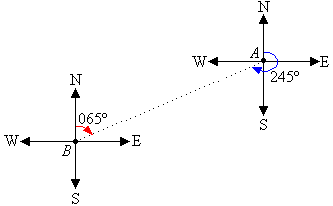###### Note:
• Three figures are used to give bearings.
• All bearings are measured in a horizontal plane.

#### Example 10

State the bearing of the point P in each of the following diagrams: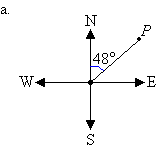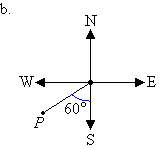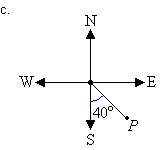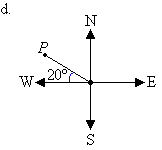##### Solution:

a.  Mark the angle in a clockwise direction by indicating the turn between the north line and the line joining the centre of the compass to the point P.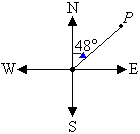The bearing of point P is 048°.

b.  Mark the angle in a clockwise direction by indicating the turn between the north line and the line joining the centre of the compass to the point P.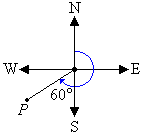The cardinal point S corresponds to 180°.  It is clear from the diagram that the required angle is 60° larger than 180°.  So, the angle measured in a clockwise direction from the north line to the line joining the centre of the compass to point P is 180° + 60° = 240°.

So, the bearing of point P is 240°.

c.  Mark the angle in a clockwise direction by indicating the turn between the north line and the line joining the centre of the compass to the point P.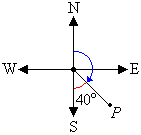The cardinal point S corresponds to 180°.  It is clear from the diagram that the required angle is 40° less than 180°.  So, the angle measured in a clockwise direction from the north line to the line joining the centre of the compass to point P is 180° 40° = 140°.

So, the bearing of point P is 140°.

d.  Mark the angle in a clockwise direction by indicating the turn between the north line and the line joining the centre of the compass to the point P.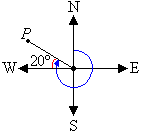The cardinal point W corresponds to 270°.  It is clear from the diagram that the required angle is 20° larger than 270°.  So, the angle measured in a clockwise direction from the north line to the line joining the centre of the compass to point P is 270° + 20° = 290°.

So, the bearing of point P is 290°.

### Direction

The conventional bearing of a point is stated as the number of degrees east or west of the north-south line.  We will refer to the conventional bearing simply as the direction.

To state the direction of a point, write:

• N or S which is determined by the angle being measured
• the angle between the north or south line and the point, measured in degrees
• E or W which is determined by the location of the point relative to the north-south line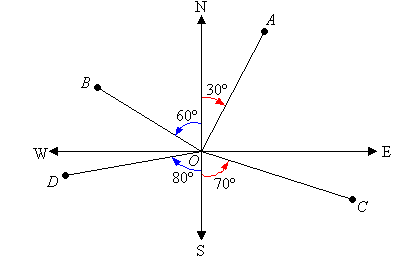E.g. In the above diagram, the direction of:

• A from O is N30ºE.
• B from O is N60ºW.
• C from O is S70ºE.
• D from O is S80ºW.
###### Note:

N30ºE means the direction is 30º east of north.

#### Example 11

Describe each of the following bearings as directions.
a.  076°
b.  150°
c.  225°
d.  290°

##### Solution:

a.  The position of a point P on a bearing of 076° is shown in the following diagram.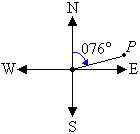The position of the point P is 76° east of north.  So, the direction is N76°E.

b.  The position of a point P on a bearing of 150° is shown in the following diagram.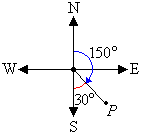The position of the point P is 180° 150° = 30° east of south.  So, the direction is S30°E.

c.  The position of a point P on a bearing of 225° is shown in the following diagram.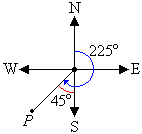The position of the point P is 225° 180° = 45° west of south.  So, the direction is S45°W.

d.  The position of a point P on a bearing of 290° is shown in the following diagram.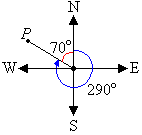The position of the point P is 360° 290° = 70° west of north.  So, the direction is N70°W.

###### Key Terms

Copyright © 2000-2020 mathsteacher.com Pty Ltd.  All rights reserved.
Australian Business Number 53 056 217 611

Copyright instructions for educational institutions

Please read the Terms and Conditions of Use of this Website and our Privacy and Other Policies.
If you experience difficulties when using this Website, tell us through the feedback form or by phoning the contact telephone number.